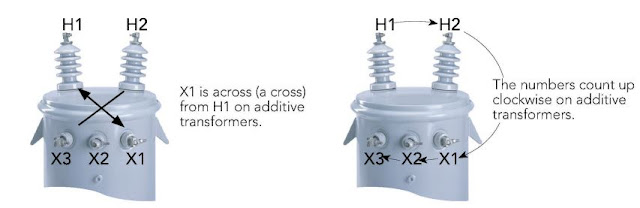Photo Credit: Electric Power Inc

An understanding of polarity is essential to correctly construct three-phase transformer banks and to properly parallel single or three-phase transformers with existing electrical systems. A knowledge of polarity is also required to connect potential and current transformers to power metering devices and protective relays. The basic theory of additive and subtractive polarity is the underlying principle used in step voltage regulators where the series winding of an autotransformer is connected to either buck or boost the applied line voltage.

When you have a transformer, it can be either additive or subtractive with regard to polarity. What determines this is the setup of the secondary coil in the transformer.

• If the secondary coil is wound around the core in the opposite direction as the primary coil, than the transformer is said to be Additive.
• If the secondary coil is wound around the core in the same direction as the primary coil, than the transformer is said to be Subtractive.

When looking at an additive transformer, the secondary bushings are numbers from right to left (X3, X2, and X1) See the diagram below for a visualization. One way to identify that you are looking at an additive transformer is to read the bushings clockwise.

### Related Article: What are the Basic Characteristics of an Ideal Transformer?

Starting on the top left primary bushing, the numbers should count up. (H1, H2, X1, X2, X3). Once again see below for a visualization of this concept.Additive Polarity

### Subtractive Transformers

Think of these as the opposite of additive transformers. The secondary bushings are numbers from left to right (X1, X2, and X3). See the diagram below for a visualization. One way to remember this is by the phrase "Same side subtractive", meaning that if the H1 primary bushing and X1 secondary bushing are on the same side then it is a subtractive transformer. See the diagram below for this concept.

### Current Direction relative to Polarity

Polarity in transformers stems back to the iron core and the windings that connect to the source and load side. If the primary and secondary coils on a transformer are wound in the same direction, than the secondary current flows in the same direction as the primary current, hence why the H1 and X1 are on the same side of the transformer.

If the primary and secondary coils of the transformer are wound in the opposite direction, than the secondary current flows in the opposite direction as the primary current, hence why the H1 and X1 are on opposite sides (diagonal from each other).

This concept is simple but always gets you to the right answer.  So when you are looking at a transformer, always remember this fact. In the example of an additive transformer, where the H1 and X3 are on the same side, everything flows from H1 to X1, despite them being on opposite sides. Don't make the silly mistake that what goes into H1 must come out on the same side as X3.

### Polarity Test

In situations where the secondary bushing identification is not available or when a transformer has been rewound, it may be necessary to determine the transformer polarity by test. The following procedure can be used.

The H1 (left-hand) primary bushing and the left-hand secondary bushing are temporarily jumpered together and a test voltage is applied to the transformer primary. The resultant voltage is measured between the right-hand bushings. If the measured voltage is greater than the applied voltage, the transformer is Additive Polarity because the polarity is such that the secondary voltage is being added to the applied primary voltage. If, however, the measured voltage across the right-hand bushings is less than the applied primary voltage, the transformer is Subtractive Polarity.

Note: For safety and to avoid the possibility of damaging the secondary insulation, the test voltage applied to the primary should be at a reduced voltage and should not exceed the rated secondary voltage.

In the example below, if the transformer is actually rated 480 - 120 volts, the transformer ratio is 4:1 (480 / 120 = 4). Applying a test voltage of 120 volts to the primary will result in a secondary voltage of 30 volts (120 / 4 = 30). If transformer is subtractive polarity, the voltmeter will read 90 volts (120 - 30 = 90). If the voltmeter reads 150 volts, the transformer is additive polarity (120 + 30 = 150).

The red arrows indicate the relative magnitude and direction of the primary and secondary voltages.Transformer Polarity Test

Reference:
• Transformer Polarity
• Publisher: Kilowatt Classroom, LLC, 2012
1.